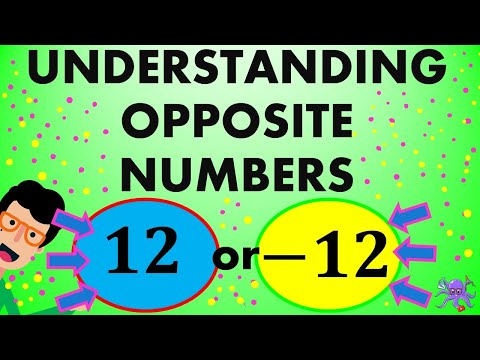# Calculate the opposite of 1 6/12

Use our calculator to get the opposite of 1 6/12. Also, learn what is an opposite of a number as use our step-by-step calculator to find the opposite of any real number or fraction.

### Opposite of a Number or of a Fraction Calculator

Ex.: 2, -2.33, -1/2, 2 5/3, etc.
Note that 2 5/3 means one and a half = 2 + 5/3

Click here to calculate the inverse or reciprocal of 1 6/12.

## What Does the Opposite of a Number Mean?

Definition 1: Opposite number or additive inverse of any number (n) is a number which, if added to , results in 0, the identity element of addition. The opposite number for n is written as −n.

Definition 2: The opposite of a number is its additive inverse, which means that its sign is reversed. A number and its additive inverse equal zero when added.

Definition 3: The opposite of a number is the number on the other side of the 0 number line, and the same distance from 0. Examples:

The opposite of 3 is -3 and vice-versa.## Video on How To Find The Oposite of Whole Numbers, Fractions and Decimal Numbers

This excellent video explains how to find the reciprocal of a whole number, a fraction, as well as, the reciprocal of a mixed number.↼ Click on the image to watch the video.

### Disclaimer

While every effort is made to ensure the accuracy of the information provided on this website, neither this website nor its authors are responsible for any errors or omissions. Therefore, the contents of this site are not suitable for any use involving risk to health, finances or property.